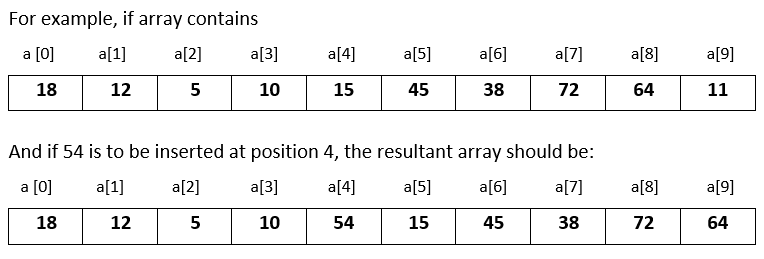# Input 10 numbers in 1d array and insert a number at the given position in Java

###### One Dimensional Array - Question 10

In this question, we will see how how to input 10 numbers in a one dimensional integer array and insert a number at a given position in the array in Java programming. To know more about one dimensional array click on the one dimensional array lesson.

Q10) Write a program in Java to input 10 numbers in a one dimensional integer array and input a number and a position. Now insert the number at that position by shifting the rest of the numbers to the right. The last element is therefore removed from the array.#### Program

``````import java.util.Scanner;

public class Q10
{
public static void main(String args[])
{
int a[]=new int, i,n,p;
Scanner sc=new Scanner(System.in);

System.out.println("Enter 10 numbers");
for(i=0; i<10; i++)
{
a[i]=sc.nextInt();
}

System.out.println("Enter number and its position to insert in the array");
n=sc.nextInt();
p=sc.nextInt();
for(i=9; i>p; i--)
{
a[i]=a[i-1];
}
a[i]=n;

System.out.println("\nModified array after inserting the number");
for(i=0; i<10; i++)
{
System.out.print(a[i]+" ");
}
}
}``````

#### Output

```Enter 10 numbers
18
12
5
10
15
45
38
72
64
11
Enter number and its position to insert in the array
54
4

Modified array after inserting the number
18 12 5 10 54 15 45 38 72 64```